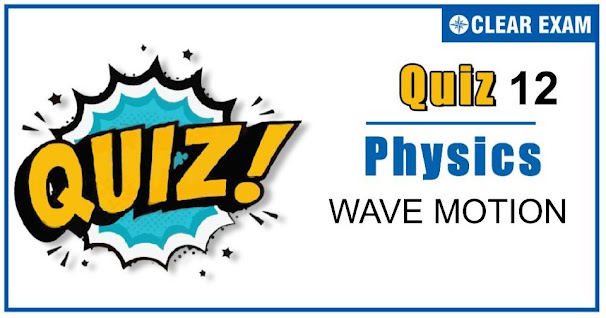## [LATEST]\$type=sticky\$show=home\$rm=0\$va=0\$count=4\$va=0

As per analysis for previous years, it has been observed that students preparing for NEET find Physics out of all the sections to be complex to handle and the majority of them are not able to comprehend the reason behind it. This problem arises especially because these aspirants appearing for the examination are more inclined to have a keen interest in Biology due to their medical background. Furthermore, sections such as Physics are dominantly based on theories, laws, numerical in comparison to a section of Biology which is more of fact-based, life sciences, and includes substantial explanations. By using the table given below, you easily and directly access to the topics and respective links of MCQs. Moreover, to make learning smooth and efficient, all the questions come with their supportive solutions to make utilization of time even more productive. Students will be covered for all their studies as the topics are available from basics to even the most advanced.

Q1. If the amplitude of sound is doubled and the frequency reduced to one-fourth, the intensity of sound at the same point will be
•  Increased by a factor of 2
•  Decreased by a factor of 2
•  Decreased by a factor of 4
•  Unchanged
Solution
I=2π2 a2 n2 vρ⇒I∝a2 n2 ⇒I1/I2 =(a_1/a_2 )2×(n1/n2)2=(1/2)2×(1/(1/4))2 ⇒I2=I1/4

Q2.When two sinusoidal waves moving at right angles to each other superimpose, they produce
•  Beats
•  Interface
•  Stationary waves
•  Lissajous figure
Solution
If two SHMs act in perpendicular direction, then their resultant motion is in the form of a straight line or a circle or a parabola etc, depending on the frequency ratio of the two SHMs and their phase difference. These figures are Lissajous figure.

Q3.  Two stretched strings of same material are vibrating under same tension in fundamental mode. The ratio of their frequencies is 1 : 2 and ratio of the length of the vibrating segments is 1 : 4. Then the ratio of the radii of the strings is
•   2:1
•  4:1
•  3:2
•  8:1
Solution
Frequency f=1/2L √(T/M)=1/2L √(T/(πr2 (1)ρ)) =1/2rL √(T/πρ)⇒f1/f2 =(r2/r1 )(L2/L1 )⇒1/2=(r2/r1 )(4/1) ⇒r2/r1 =1/8⇒r1/r2 =8/1

Q4. A 20 cm long string, having a mass of 1.0 g, is fixed at both the ends. The tension in the string is 0.5 N. the string is set into vibration using an external vibrator of frequency 100 Hz. Find the separation (in cm) between the successive nodes on the string
•  5
•  6
•  2
•  3/2
Solution
Distance between the successive nodes, d=λ/2 =v/2f =√(T/μ)/2f Substituting the value we get D=5cm

Q5.The equation of a cylindrical progressive wave is
•  y=a sin⁡ωt
•  y=a sin⁡(ωt-kr)
•  y=a/√r sin⁡(ωt-kr )
•  y=a/r sin⁡(ωt-kr)
Solution
The amplitude of a plane progressive wave=a, that of a cylindrical progressive wave is a/√r.

Q6. The sound carried by air from a sitar to a listener is a wave of the following type
•  Longitudinal stationary
•  Transverse progressive
• Transverse stationary
•  Longitudinal progressive
Solution
Observer receives sound waves (music) which are longitudinal progressive waves

Q7.The line of a sight of a jet plane makes an angle of 〖60〗0 with the vertical, and the sound appears to be coming from over the head of the observer. The speed of jet plane is (taking speed of sound waves to be v)
•  v
•  v/√3
•  v√3
•  2v
Solution
Proceeding as in above question, tan⁡〖θ=tan⁡〖60°=vp/v〗 〗 ∴vp=v×tan⁡〖60°=v√3〗

Q8.A spherical source of power 4 W and frequency 800 Hz is emitting sound waves. The intensity of waves at a distance 200 m is
•  8×〖10〗(-6)W/m2
•  T2×〖10〗(-4) W/m2
•  1×〖10〗(-4) W/m2
•  4 W/m2
Solution
Intensity =Power/Area=4/(4π×(200)2 )=7.9×〖10〗(-6) W/m2

Q9. The velocity of waves in a string fixed at both ends is 2 m/s. The string forms standing waves with nodes 5.0 cm apart. The frequency of vibration of the string in Hz is
•  40
•  30
•  20
•  10
Solution
Here λ/2=5.0 cm ⇒λ=10 cm Hence n=v/λ=200/10=20 Hz

Q10. ‘SONAR’ emits which of the following waves
•  Ultrasonic waves
•  Light waves
• Magnetic waves
Solution
SONAR emits ultrasonic waves## Want to know more

Please fill in the details below:

## Latest NEET Articles\$type=three\$c=3\$author=hide\$comment=hide\$rm=hide\$date=hide\$snippet=hide

Name

ltr
item
BEST NEET COACHING CENTER | BEST IIT JEE COACHING INSTITUTE | BEST NEET & IIT JEE COACHING